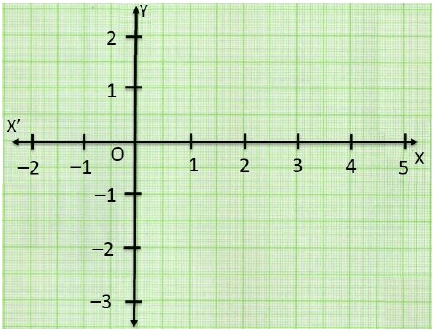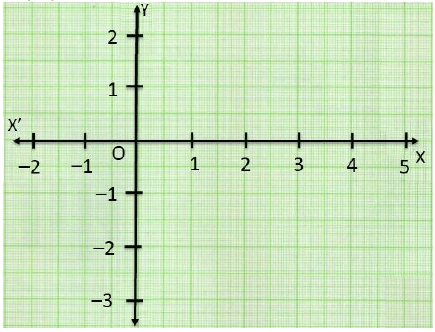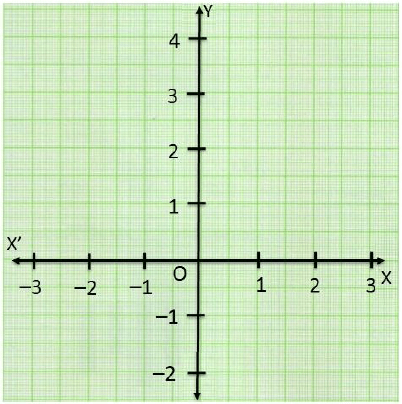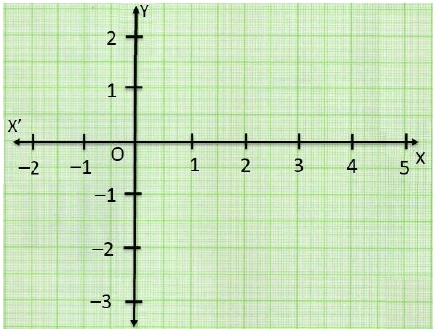Test: Coordinate Geometry- Assertion & Reason Type Questions

# Test: Coordinate Geometry- Assertion & Reason Type Questions - Class 8

Test Description

## 10 Questions MCQ Test Advance Learner Course: Mathematics (Maths) Class 8 - Test: Coordinate Geometry- Assertion & Reason Type Questions

Test: Coordinate Geometry- Assertion & Reason Type Questions for Class 8 2023 is part of Advance Learner Course: Mathematics (Maths) Class 8 preparation. The Test: Coordinate Geometry- Assertion & Reason Type Questions questions and answers have been prepared according to the Class 8 exam syllabus.The Test: Coordinate Geometry- Assertion & Reason Type Questions MCQs are made for Class 8 2023 Exam. Find important definitions, questions, notes, meanings, examples, exercises, MCQs and online tests for Test: Coordinate Geometry- Assertion & Reason Type Questions below.
Solutions of Test: Coordinate Geometry- Assertion & Reason Type Questions questions in English are available as part of our Advance Learner Course: Mathematics (Maths) Class 8 for Class 8 & Test: Coordinate Geometry- Assertion & Reason Type Questions solutions in Hindi for Advance Learner Course: Mathematics (Maths) Class 8 course. Download more important topics, notes, lectures and mock test series for Class 8 Exam by signing up for free. Attempt Test: Coordinate Geometry- Assertion & Reason Type Questions | 10 questions in 20 minutes | Mock test for Class 8 preparation | Free important questions MCQ to study Advance Learner Course: Mathematics (Maths) Class 8 for Class 8 Exam | Download free PDF with solutions
 1 Crore+ students have signed up on EduRev. Have you?
Test: Coordinate Geometry- Assertion & Reason Type Questions - Question 1

### DIRECTION : In the following questions, a statement of assertion (A) is followed by a statement of reason (R). Mark the correct choice as:Assertion : The point (0, 4) lies on y -axis.Reason : The x coordinate on the point on y -axis is zero.

Detailed Solution for Test: Coordinate Geometry- Assertion & Reason Type Questions - Question 1

We know that the if the point lies on y-axis, its x-coordinate is 0.

So, Reason is correct.

The x coordinate of the point (0, 4) is zero.

So, Point (0, 4) lies on y -axis.

So, Assertion is also correct Correct option is (a) Both assertion (A) and reason (R) are true and reason (R) is the correct explanation of assertion (A).

Test: Coordinate Geometry- Assertion & Reason Type Questions - Question 2

### DIRECTION : In the following questions, a statement of assertion (A) is followed by a statement of reason (R). Mark the correct choice as:Assertion: Point (4, -2) lies in IV quadrant.Reason: The perpendicular distance of a point from the y-axis is called its abscissa.

Detailed Solution for Test: Coordinate Geometry- Assertion & Reason Type Questions - Question 2We know that the perpendicular distance of a point from y-axis is called its x-coordinate or abscissa.

So, Reason is correct.

Point (4, -2) lies in IV quadrant.

So, Assertion is also correct but Reason is not the correct explanation of Assertion. Correct option is (b) Both assertion (A) and reason (R) are true and reason (R) is not the correct explanation of assertion (A).

Test: Coordinate Geometry- Assertion & Reason Type Questions - Question 3

### DIRECTION : In the following questions, a statement of assertion (A) is followed by a statement of reason (R). Mark the correct choice as:Assertion : If the ordinate of a point is equal to its abscissa, then the point lies either in the first quadrant or in the second quadrant.Reason : A point both of whose coordinates are negative will lie in third quadrants.

Detailed Solution for Test: Coordinate Geometry- Assertion & Reason Type Questions - Question 3
We know that A point both of whose coordinates are negative will lie in third quadrants. So, Reason is correct.

Also we know that If the ordinate of a point is equal to its abscissa, then the point lies either in the first quadrant or in the third quadrant. So, Assertion is not correct

Correct option is (d) Assertion (A) is false but reason (R) is true.

Test: Coordinate Geometry- Assertion & Reason Type Questions - Question 4

DIRECTION : In the following questions, a statement of assertion (A) is followed by a statement of reason (R). Mark the correct choice as:

Assertion: Point A(-2, -4) lies on III quadrant

Reason: A point both of whose coordinates are negative lies in III quadrant

Detailed Solution for Test: Coordinate Geometry- Assertion & Reason Type Questions - Question 4
We know that a point both of whose coordinates are negative lies in III quadrant

So, Reason is correct.

Hence, Point A(-2, -4) lies on III quadrant So, Assertion is also correct and Reason explains Assertion

Correct option is (a) Both assertion (A) and reason (R) are true and reason (R) is the correct explanation of assertion (A).

Test: Coordinate Geometry- Assertion & Reason Type Questions - Question 5

DIRECTION : In the following questions, a statement of assertion (A) is followed by a statement of reason (R). Mark the correct choice as:

Assertion: A point whose abscissa is 2 and ordinate is -3 lies in fourth quadrant

Reason: Points of the type (–, +) lie in the second quadrant.

Detailed Solution for Test: Coordinate Geometry- Assertion & Reason Type Questions - Question 5

We know that Points of the type (–, +) lie in the second quadrant. So, Reason is correct.

Also, we know that Points of the type (+, –) lie in the fourth quadrant. Hence, point whose abscissa is 2 and ordinate is -3 lies in fourth quadrant So, Assertion is also correct but Reason is the not the correct explanation of Assertion.

Correct option is (b) Both assertion (A) and reason (R) are true but reason (R) is not the correct explanation of assertion (A).

Test: Coordinate Geometry- Assertion & Reason Type Questions - Question 6

DIRECTION : In the following questions, a statement of assertion (A) is followed by a statement of reason (R). Mark the correct choice as:

Assertion: The abscissa of a point (5, 2) is 5.

Reason: The perpendicular distance of a point from y-axis is called its abscissa.

Detailed Solution for Test: Coordinate Geometry- Assertion & Reason Type Questions - Question 6We know that the perpendicular distance of a point from y-axis is called its x-coordinate or abscissa.

So, Reason is correct.

The x co-ordinate of the point (5, 2) is 5.

So, Assertion is also correct

Correct option is (a) Both assertion (A) and reason (R) are true and reason (R) is the correct explanation of assertion (A).

Test: Coordinate Geometry- Assertion & Reason Type Questions - Question 7

DIRECTION : In the following questions, a statement of assertion (A) is followed by a statement of reason (R). Mark the correct choice as:

Assertion : The point (-2, 0) lies on y -axis and (0, 4) on x -axis.

Reason : Every point on the x -axis has zero distance from x -axis and every point on the y -axis has zero distance from y -axis.

Detailed Solution for Test: Coordinate Geometry- Assertion & Reason Type Questions - Question 7We know that Every point on the x -axis has zero distance from x -axis and every point on the y -axis has zero distance from y -axis. So, Reason is correct.

Now, point (-2, 0) lies on x-axis and (0, 4) on y-axis So, Assertion is not correct Correct option is (d) Assertion (A) is false but reason (R) is true.

Test: Coordinate Geometry- Assertion & Reason Type Questions - Question 8

DIRECTION : In the following questions, a statement of assertion (A) is followed by a statement of reason (R). Mark the correct choice as:

Assertion : The points (-1, 2) and (2,- 1) are at different positions in the coordinate plane.

Reason : Point (-1, 2) lies in II-quadrant and (2,- 1) lies in IV quadrant

Detailed Solution for Test: Coordinate Geometry- Assertion & Reason Type Questions - Question 8We know that Point (-1, 2) lies in II-quadrant and (2,- 1) lies in IV quadrant So, Reason is correct.

Hence the points (-1, 2) and (2,- 1) are at different positions in the coordinate plane. So, Assertion is also correct Correct option is (a) Both assertion (A) and reason (R) are true and reason (R) is the correct explanation of assertion (A).

Test: Coordinate Geometry- Assertion & Reason Type Questions - Question 9

DIRECTION : In the following questions, a statement of assertion (A) is followed by a statement of reason (R). Mark the correct choice as:

Assertion: The perpendicular distance of the point A(3, 4) from the y-axis is 4

Reason: The perpendicular distance of a point from y-axis is called its x-coordinate.

Detailed Solution for Test: Coordinate Geometry- Assertion & Reason Type Questions - Question 9

We know that the perpendicular distance of a point from y-axis is called its x-coordinate or abscissa.

So, Reason is correct.

The x co-ordinate of the point (3, 4) is 3.

So, Assertion is not correct Correct option is (d) Assertion (A) is false but reason (R) is true.

Test: Coordinate Geometry- Assertion & Reason Type Questions - Question 10

DIRECTION : In the following questions, a statement of assertion (A) is followed by a statement of reason (R). Mark the correct choice as:

Assertion: A point whose abscissa is -3 and ordinate is 2 lies in second quadrant

Reason: Points of the type (–, +) lie in the second quadrant.

Detailed Solution for Test: Coordinate Geometry- Assertion & Reason Type Questions - Question 10

We know that Points of the type (–, +) lie in the second quadrant. So, Reason is correct.

Hence, point whose abscissa is -3 and ordinate is 2 lies in second quadrant So, Assertion is also correct and Reason explains Assertion Correct option is (a) Both assertion (A) and reason (R) are true and reason (R) is the correct explanation of assertion (A).

## Advance Learner Course: Mathematics (Maths) Class 8

13 videos|62 docs|24 tests
Information about Test: Coordinate Geometry- Assertion & Reason Type Questions Page
In this test you can find the Exam questions for Test: Coordinate Geometry- Assertion & Reason Type Questions solved & explained in the simplest way possible. Besides giving Questions and answers for Test: Coordinate Geometry- Assertion & Reason Type Questions, EduRev gives you an ample number of Online tests for practice

## Advance Learner Course: Mathematics (Maths) Class 8

13 videos|62 docs|24 tests